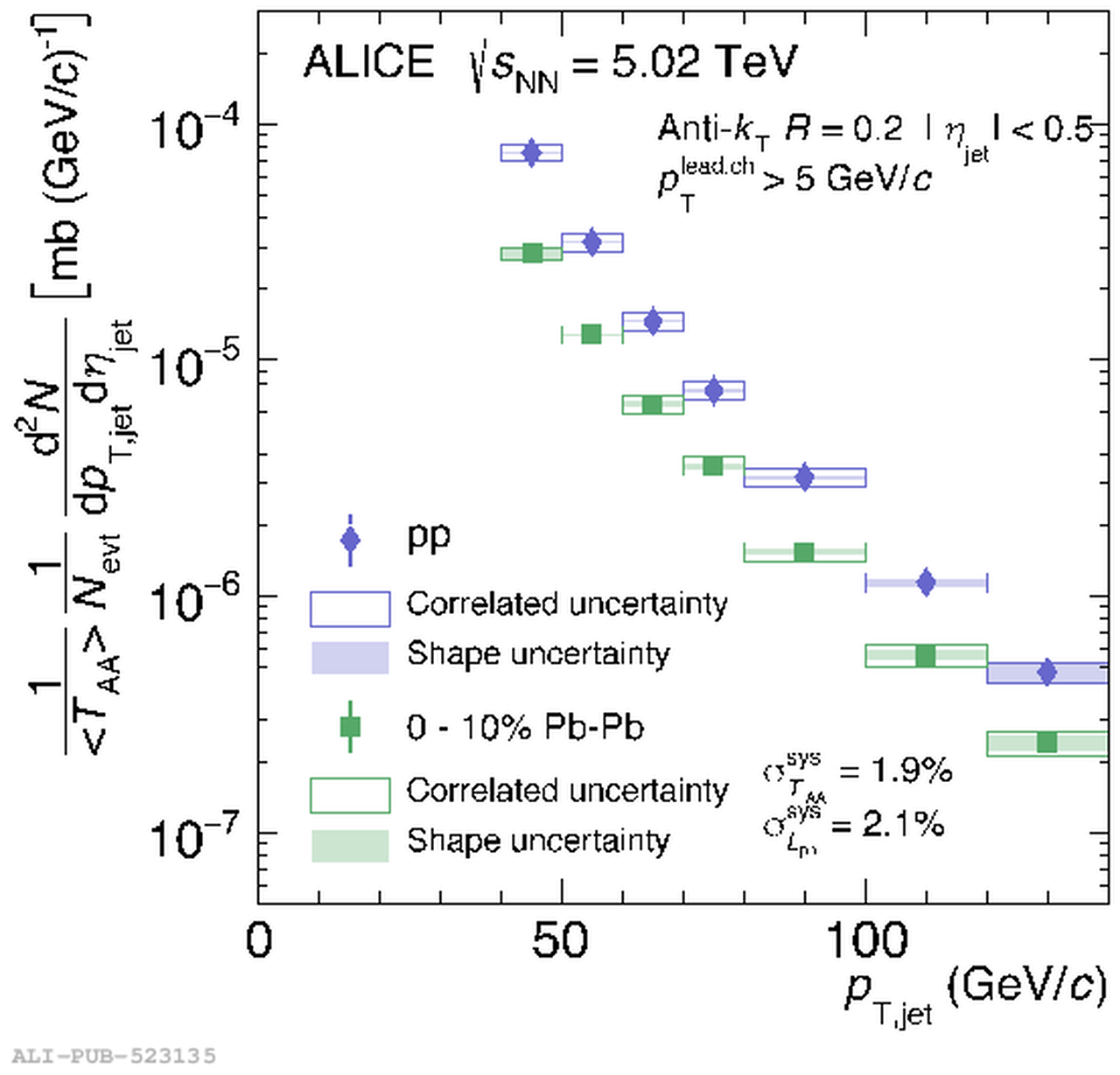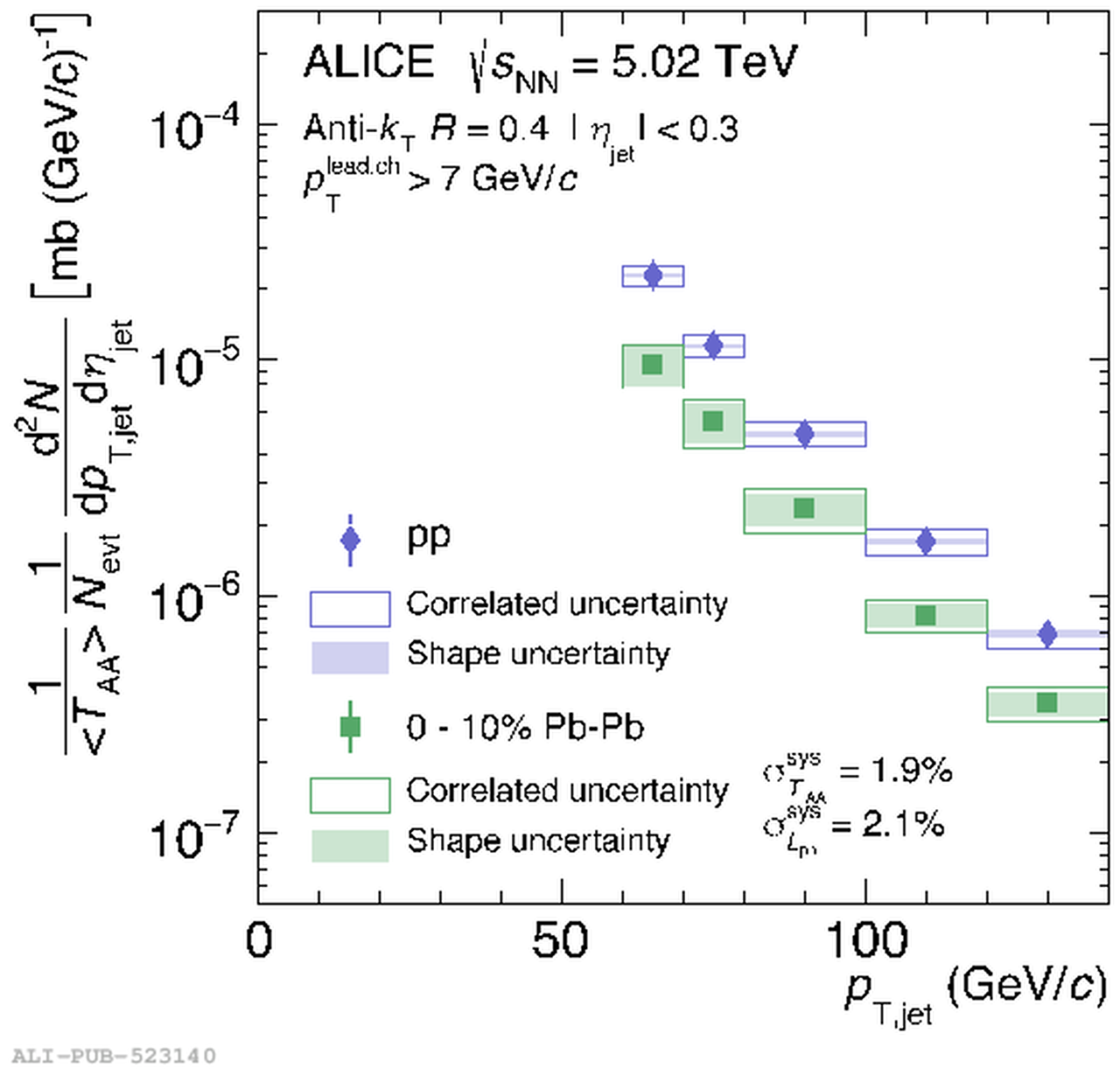# Figure 4

 Unfolded \pp{} and \PbPb{} full jet spectra at $\sqrts=5.02$ TeV for $R=0.2$ (left), with 5 \GeVc{} leading track requirement, and $R=0.4$ (right), with 7 \GeVc{} leading track requirement The \pp{} data points correspond to $\frac{\mathrm{d}^{2}\sigma}{\mathrm{d}\pTjet \mathrm{d}\etajet}$.